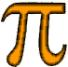# Statistics

### Statistics for university or HBOPrivate tutoring is very beneficial to students who often look up to Statistics. A highly experienced teacher who is aware of their problems can help them to get a sufficient grade, as their reviews show.

#### Statistics is a major obstacle

For many HBO and University students, Statistics, like Mathematics, is a major obstacle. This is especially the case for students in Alpha sciences. Many of these students are forced to stop their studies because they fail to pass Statistics. For this reason, many students in the Alpha sciences (Universities in Rotterdam, Tilburg, Leiden and Amsterdam) look for help in preparing statistical exams.

#### Don’t get scared by mathematical formulas!

Good knowledge of Mathematics helps to understand Statistics. But also with less mathematical knowledge, a good grade for the subject of Statistics is certainly possible. Private tutoring by an experienced lecturer is often indispensable. There is no reason for a student to be scared by complicated formulas. Private tutoring is a mean to understand this.

#### Statistics can be explained

All subjects from Statistics can be explained comprehensively. Understanding is more important than learning by heart. It is possible to explain what the various concepts of descriptive statistics mean: what is meant by arithmetic mean and median, variance and standard deviation, correlation and covariance. Of course, it is necessary to explain how to calculate these (formulas).
Various probability distribution functions such as the binomial distribution, the Poisson distribution and the Normal distribution can be explained, but also the difference between, for example, t-and z-distribution, and the application of Chi2 or F-distributions. Subjects such as hypothesis testing, ANOVA and (multiple) regression can be explained and understood without thorough knowledge of Mathematics.

#### Common sense and commitment: a guarantee for success!

Most students are bright people for whom Statistics is no ‘bridge too far’. Private tutoring and commitment (hard work) proved to be a guarantee for success!